# Introduction to the Variational Quantum Eigensolver

## Introduction

The variational quantum eigensolver (VQE) is a hybrid quantum-classical algorithm that performs the hard computation on a quantum computer then uses a classical computer to process the measurement results from the quantum computer.

The idea is quite simple: the premise of quantum computers is that the Hilbert space is so huge that we can’t reasonably explore it efficiently on a classical computer (under certain conditions - see clifford circuits) so we use a quantum computer to do the exploration.
But once we have the result from the quantum computer, we use a classical optimizer to drive us towards the solution, which in our case is the ground state of the system we are studying.

The sections that follow are all about making sense of the two paragraphs above and seeing VQE in action.

### Prerequisites

It is assumed that the reader has some basic knowledge of quantum computation. That is the reader knows what a circuit is, and what gates and qubits are. In the tooling section, theoretical tools subsection we list the necessary theoretical tools needed to make fruitful use of this tutorial.

This tutorial is very much practical oriented. I do not try to help build any intuition about VQE. The reader is encouraged to read Michał Stęchły’s blog post on VQE for a more intuitive explanation.

### Notation

We will work mostly with Pauli matrices. (The identity matrix is not a Pauli matrix but we need to list it here.) We will adopt the following notation for those matrices.

• Identity $I$ matrix:
$\sigma^{(i)} = I = \begin{bmatrix} 1 & 0 \\ 0 & 1 \end{bmatrix}$
• Pauli $X$ matrix:
$\sigma^{(x)} = X = \begin{bmatrix} 0 & 1 \\ 1 & 0 \end{bmatrix}$
• Pauli $Y$ matrix:
$\sigma^{(y)} = Y = \begin{bmatrix} 0 & -i \\ i & 0 \end{bmatrix}$
• Pauli $Z$ matrix:
$\sigma^{(z)} = Z = \begin{bmatrix} 1 & 0 \\ 0 & -1 \end{bmatrix}$
Different roles of Pauli matrices
It is important to remain cognizant of the fact that Pauli matrices can either be gates or observables. And the way they are handled in code depends on whether one is dealing with a gate or an observable.
For instance, as a gate the Pauli matrix $\sigma^{(x)}$ will appear exactly as it is in both code and equations. But as an observable, it will appear as the matrix above in equations but will be translated to a Hadamard gate in code.

In addition to Pauli matrices, we will make use of the following quantum gates:

$H = \dfrac{1}{\sqrt{2}}\begin{bmatrix} 1 & 1 \\ 1 & -1 \end{bmatrix}$
• Phase gate:
$S = \begin{bmatrix} 1 & 0 \\ 0 & i \end{bmatrix}$
• Phase shift gate:
$P(\phi) = \begin{bmatrix} 1 & 0 \\ 0 & {\rm e}^{i\phi} \end{bmatrix}$
$RX(\theta) = \begin{bmatrix} \cos\frac{\theta}{2} & -i\sin\frac{\theta}{2} \\ -i\sin\frac{\theta}{2} & \cos\frac{\theta}{2} \end{bmatrix}$
$RY(\theta) = \begin{bmatrix} \cos\frac{\theta}{2} & -\sin\frac{\theta}{2} \\ \sin\frac{\theta}{2} & \cos\frac{\theta}{2} \end{bmatrix}$

### Organization of the tutorial

In the next section we introduce the tools (theoretical and practical) needed to understand and implement VQE.

In the basic theory section, we justify why VQE works and how it works. We will provide the physical justification of the algorithm and explore two simple examples from which we can build up more complicated examples.

In the ansatz design section we learn about the different ways ansätze are designed. We will explore some of the challenges that occur with particular choices of different designs.

In the optimizer selection section we talk about the different optimizers that are available so the reader can play with them.

In the measurement reduction section we show that it is possible to reduce the number of measurements so we can obtain results faster.

And last in the practical considerations section we think about the limitations of VQE.

## Tooling

### Theoretical tools

The reader is expected to know the following mathematics:

1. Finding the eigenvalues and eigenvectors of a matrix.
2. Basic operations on matrices.
3. Kronecker product, especially using the mixed-product property.

The reader is also expected to know the following basic quantum computation theory:

1. Quantum states as rays in Hilbert space.
2. Quantum gates as evolution operators of quantum states.

A review of the measurement postulate will be provided so it is not a prerequisite.

The book Quantum Computation and Quantum Information (Nielsen & Chuang, 2010) has the necessary background that is required for much of this post.

### Software tools

We will use PennyLane from Xanadu to run code we write. It is an easy to use library and has an excellent documentation which includes demos, tutorials and reference documentation.

The installation instructions can be found at https://pennylane.ai/install/.

Note: we will use the noiseless state vector simulator so as not worry our heads with the complications that come with having a noisy device. Maybe a future post will explore the behavior of VQE when noise is taken into account.

## Basic theory

In physics, if we know the Hamiltonian of a system then we know the dynamics of the system. This can be easily seen by looking at Schrödinger’s equation. Therefore, we will first need to have our problem encoded in a Hamiltonian.

We won’t spend our time trying to devise Hamiltonians — this is very hard work — but we will assume that they are given to us. Our goal will simply be finding the ground state energy (zero-point energy) of the given Hamiltonian.

We care about the ground state energy because it corresponds to the energy of any system close to absolute zero. It is true that sometimes we care about excited states of a system but in this tutorial we won’t worry about that.

We begin by justifying why VQE works. Then we see why a classical optimizer is necessary to find the ground state energy. Having grounded ourselves – pun intended – we tie all the different parts required to make VQE work in some sort of template. Last, we code a couple of examples to see if simulations match theoretical predictions.

### The measurement postulate

Let $H$, the Hamiltonian, be the observable representing the total energy of the system. By the spectral theorem $H$ has spectral decomposition:

$H = \sum_{i} \lambda_i P_i \tag{1}$

Where $\lambda_i$ is an eigenvalue and $P_i$ is a projector onto the eigenspace of $H$ with corresponding eigenvalue $\lambda_i$. That simply means that $P_{i}^{2} = P_i$ and $P_i = \ket{\lambda_i} \bra{\lambda_i}$ where $\{ \ket{\lambda_i} \}$ is the eigenspace of $H$ with each eigenvector $\ket{\lambda_i}$ associated to eigenvalue $\lambda_i$.

We can therefore write equation $(\href{#mjx-eqn:1}{1})$ as:

$H = \sum_{i} \lambda_i \ket{\lambda_i} \bra{\lambda_i} \tag{1'}$

Upon measurement (before the measurement but not after) the probability of measuring the eigenvalue $\lambda_i$ given some state $\ket{\psi}$ is given by:

$p(\lambda_i) = \bra{\psi} P_i \ket{\psi} = \braket{\psi\\|\lambda_i} \braket{\lambda_i\\|\psi} = \braket{\psi\\|\lambda_i} \braket{\psi\\|\lambda_i}^* = \lvert\braket{\psi\\|\lambda_i}\rvert^{2} \tag{2}$

The final state after measurement is given by:

$\ket{\psi} \mapsto \frac{P_i \ket{\psi}}{\sqrt{p(\lambda_i)}}$

We will care only about post-measurement states for the purpose of calculating probabilities but not much else beside that.

Measuring observables and eigenvalues
It is important to realize that when we are asked to measure an observable, we are being asked to find the probabilities of measuring its eigenvalues given some state.
In practice though we will see the eigenvectors appearing with a certain frequency. So we will calculate the probability from those frequencies.
Different terminology for measurements
It is common to hear/read that a measurement was performed in a certain basis.
Let us recall that the eigenvectors of a Hermitian operator form a complete basis. This means that we can express any state in the corresponding space as a linear combination of the eigenvectors of that Hermitian operator.
For example the $\sigma^{(z)}$ observable, being a Hermitian operator, has eigenvectors $\ket{0} = \begin{bmatrix}1\\0\end{bmatrix}$ and $\ket{1} = \begin{bmatrix}0\\1\end{bmatrix}$.
Consequently every one qubit state can be written as $\ket{\psi} = c_0 \ket{0} + c_1 \ket{1}$. States written using the eigenvectors of $\sigma^{(z)}$ are said to be written in the standard basis or simply in the $\sigma^{(z)}$ basis.
(It is called standard basis because it maps to classical binary, which is the standard for classical computing.)

Similarly the $\sigma^{(x)}$ observable has eigenvectors $\ket{+} = \dfrac{1}{\sqrt{2}}\begin{bmatrix}1\\1\end{bmatrix}$ and $\ket{-} = \dfrac{1}{\sqrt{2}}\begin{bmatrix}1\\-1\end{bmatrix}$.
Therefore every one qubit state can also be written as $\ket{\psi} = c_0 \ket{+} + c_1 \ket{-}$. States written using the eigenvectors of $\sigma^{(x)}$ are said to be written in the Hadamard basis or the simply in the $\sigma^{(x)}$ basis.
(It is called the Hadamard basis because the eigenvectors of $\sigma^{(x)}$ are obtained by applying the Hadamard gate to eigenvectors of $\sigma^{(z)}$.)

However, we will find it convenient most of the time to work in the standard basis.

So performing a measurement in the standard basis is equivalent to using projectors $P_0=\ket{0}\bra{0}$ and $P_1=\ket{1}\bra{1}$.
Equivalently, performing a measurement in the Hadamard basis is equivalent to using the projectors $P_+=\ket{+}\bra{+}$ and $P_-=\ket{-}\bra{-}$

#### Pauli matrices and the identity form a complete basis

The Pauli matrices with the identity matrix form a complete basis for all observables on a single qubit. The tensor products of Pauli matrices along with the identity matrix form a complete basis for observables on multiple qubits.

What that means is that every observables we can think about can be expressed as a linear combination of Pauli matrices and the identity matrix.
Therefore we only need to learn how to measure Pauli matrices.

#### Measurement of $\sigma^{(z)}$ with respect to $\ket{\psi} = c_0 \ket{0} + c_1 \ket{1}$

The eigenvalues and eigenvectors of $\sigma^{(z)}$ are calculated in the derivations section and are found to be:

1. Eigenvalue $+1$ with eigenvector $\ket{0} = \begin{bmatrix} 1 \\ 0\end{bmatrix}$
2. Eigenvalue $-1$ with eigenvector $\ket{1} = \begin{bmatrix} 0 \\ 1\end{bmatrix}$
• Measurement with respect to $\ket{\psi} = c_0 \ket{0} + c_1 \ket{1}$
We calculate only the probability of obtaining eigenvalue $+1$ given the state $\ket{\psi} = c_0 \ket{0} + c_1 \ket{1}$. The calculation for eigenvalue $-1$ is similar and left as an exercise.
\begin{align} p(+1) &= \lvert\braket{\psi\\|0}\rvert^{2} \\ &= \lvert (c_0 \ket{0} + c_1 \ket{1})\ket{0}\rvert^{2} \\ &= \lvert c_0 \braket{0\\|0} + c_1 \braket{1\\|0} \rvert^{2} \\ &= \lvert c_0 \rvert^{2} \\ \end{align}
• Quantum circuit for performing the measurement
The measurement of $+1$ corresponds to the use of the projector $P_+ = \ket{0}\bra{0}$. Therefore we have:
\begin{align} p(+1) &= \bra{\psi}P_+\ket{\psi} \\ &= \braket{\psi\\|0}\braket{0\\|\psi} \\ &= \braket{\psi\\|I\\|0}\braket{0\\|I\\|\psi} \\ &= \lvert \braket{0\\|I\\|\psi} \rvert^{2} \\ \end{align}

Concomitantly, the measurement of $-1$ corresponds to the use of the projector $P_- = \ket{1}\bra{1}$ leading to:

\begin{align} p(-1) &= \bra{\psi}P_-\ket{\psi} \\ &= \braket{\psi\\|1}\braket{1\\|\psi} \\ &= \braket{\psi\\|I\\|1}\braket{1\\|I\\|\psi} \\ &= \lvert \braket{1\\|I\\|\psi} \rvert^{2} \\ \end{align}

We conclude then that the circuit to perform a measurement of the $\sigma^{(z)}$ observable given a state $\psi$ is as follows: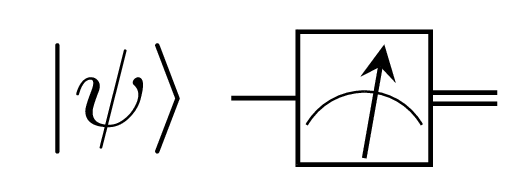Measurement of the $\sigma^{(z)}$ observable: since the identity $I$ action on the state $\ket{\psi}$ is a no-op we need not do anything, we just measure directly.
• Code for performing the measurement
We prepare the state $\ket{\psi} = RY(^\pi/_2)\ket{0}$ and measure the $\sigma^{(z)}$ observable with respect to that state. $RY$ is a rotation about the y-axis.
Measurent of the $\sigma^{(z)}$ observable: we prepare the state $\ket{\psi} = RY(^\pi/_2)\ket{0}$ as a generic example, any state would do.

Note that if we prepared eigenvectors of $\sigma^{(z)}$ we will obtain eigenvalues with $100\%$ probability. That is if we prepare the $\ket{0}$ state, we will obtain eigenvalue $+1$ with $100\%$ probability.
Equivalently, if we prepare $\ket{1}$, we will obtain eigenvalue $-1$ with $100\%$ probability.

#### Measurement of $\sigma^{(y)}$ with respect to $\ket{\psi} = c_0 \ket{0} + c_1 \ket{1}$

The eigenvalues and eigenvectors of $\sigma^{(y)}$ are calculated in the derivations section and are found to be:

1. Eigenvalue $+1$ with eigenvector $\ket{+i} = \dfrac{1}{\sqrt{2}}(\ket{0} + i\ket{1})$
2. Eigenvalue $-1$ with eigenvector $\ket{-i} = \dfrac{1}{\sqrt{2}}(\ket{0} - i\ket{1})$
• Measurement with respect to $\ket{\psi} = c_0 \ket{0} + c_1 \ket{1}$
We calculate only the probability of obtaining eigenvalue $+1$ given the state $\ket{\psi} = c_0 \ket{0} + c_1 \ket{1}$. The calculation for eigenvalue $-1$ is similar and left as an exercise.
\begin{align} p(+1) &= \lvert\braket{\psi\\|+i}\rvert^{2} \\ &= \lvert (c_0 \ket{0} + c_1 \ket{1})\ket{+i}\rvert^{2} \\ &= \lvert c_0 \braket{0\\|+i} + c_1 \braket{1\\|+i} \rvert^{2} \\ &= \left\lvert c_0 \left(\bra{0} \left(\dfrac{1}{\sqrt{2}} (\ket{0} + i\ket{1})\right)\right) + c_1 \left(\bra{1} \left(\dfrac{1}{\sqrt{2}} (\ket{0} + i\ket{1})\right)\right) \right\rvert^{2} \\ &= \left\lvert \dfrac{c_0}{\sqrt{2}}\left( \braket{0\\|0} \right) + \dfrac{ic_1}{\sqrt{2}}\left( \braket{1\\|1} \right) \right\rvert^{2} \\ &= \left\lvert \dfrac{c_0}{\sqrt{2}} + \dfrac{ic_1}{\sqrt{2}} \right\rvert^{2} \\ &= \dfrac{1}{2} \lvert c_0 + ic_1 \rvert^{2} \end{align}
• Quantum circuit for performing the measurement
The measurement of $+1$ corresponds to the use of the projector $P_+ = \ket{+i}\bra{+i}$. Therefore we have:
\begin{align} p(+1) &= \bra{\psi}P_+\ket{\psi} \\ &= \braket{\psi\\|+i}\braket{+i\\|\psi} \\ &= \braket{\psi\\|SH\\|0}\braket{0\\|HS^\dagger\\|\psi} \\ &= \lvert \braket{0\\|HS^\dagger\\|\psi} \rvert^{2} \\ \end{align}

Similarly, the measurement of $-1$ corresponds to the use of the projector $P_- = \ket{-i}\bra{-i}$ leading to:

\begin{align} p(-1) &= \bra{\psi}P_-\ket{\psi} \\ &= \braket{\psi\\|-i}\braket{-i\\|\psi} \\ &= \braket{\psi\\|SH\\|1}\braket{1\\|HS^\dagger\\|\psi} \\ &= \lvert \braket{1\\|HS^\dagger\\|\psi} \rvert^{2} \\ \end{align}

We conclude then that the circuit to perform a measurement of the $\sigma^{(y)}$ observable given a state $\psi$ is as follows: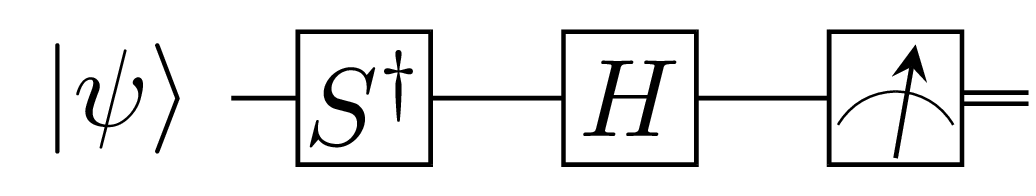Measurement of the $\sigma^{(y)}$ observable: we need to perform a basis change from the $\sigma^{(z)}$ basis to the $\sigma^{(y)}$ basis using $HS^\dagger$ then perform a standard measurement in the $\sigma^{(z)}$ basis. We will get eigenvectors in the $\sigma^{(z)}$ basis but the probabilities will correspond to measurements of the eigenvalues of $\sigma^{(y)}$.
• Code for performing the measurement
We prepare the state $\ket{\psi} = H\ket{0}$ and measure the $\sigma^{(y)}$ observable with respect to that state.
Measurement of the $\sigma^{(y)}$ observable: we prepare the state $\ket{\psi} = H\ket{0}$. We note that we obtain eigenvalue $+1$ with appromixately $0.5$ probablity and same for eigenvalue $-1$. It is easy to verify that this corresponds to theoretical predictions.
Also, note that measure() and custom_measure() provide the same results. In the first case, we use facilities provided by PennyLane. In the second case, we use the formula derived.

Note that if we prepared eigenvectors of $\sigma^{(y)}$ we will obtain eigenvalues with $100\%$ probability. That is if we prepare the $\ket{+i} = SH\ket{0}$ state, we will obtain eigenvalue $+1$ with $100\%$ probability.
Equivalently, if we prepare $\ket{-i} = SH\ket{1}$, we will obtain eigenvalue $-1$ with $100\%$ probability.

#### Measurement of $\sigma^{(x)}$ with respect to $\ket{\psi} = c_0 \ket{0} + c_1 \ket{1}$

The eigenvalues and eigenvectors of $\sigma^{(x)}$ are calculated in the derivations section and are found to be:

1. Eigenvalue $+1$ with eigenvector $\ket{+} = \dfrac{1}{\sqrt{2}}(\ket{0} + \ket{1})$
2. Eigenvalue $-1$ with eigenvector $\ket{-} = \dfrac{1}{\sqrt{2}}(\ket{0} - \ket{1})$
• Measurement with respect to $\ket{\psi} = c_0 \ket{0} + c_1 \ket{1}$
We calculate only the probability of obtaining eigenvalue $+1$ given the state $\ket{\psi} = c_0 \ket{0} + c_1 \ket{1}$. The calculation for eigenvalue $-1$ is similar and left as an exercise.
\begin{align} p(+1) &= \lvert\braket{\psi\\|+}\rvert^{2} \\ &= \lvert (c_0 \ket{0} + c_1 \ket{1})\ket{+}\rvert^{2} \\ &= \lvert c_0 \braket{0\\|+} + c_1 \braket{1\\|+} \rvert^{2} \\ &= \left\lvert c_0 \left(\bra{0} \left(\dfrac{1}{\sqrt{2}} (\ket{0} + \ket{1})\right)\right) + c_1 \left(\bra{1} \left(\dfrac{1}{\sqrt{2}} (\ket{0} + \ket{1})\right)\right) \right\rvert^{2} \\ &= \left\lvert \dfrac{c_0}{\sqrt{2}}\left( \braket{0\\|0} \right) + \dfrac{c_1}{\sqrt{2}}\left( \braket{1\\|1} \right) \right\rvert^{2} \\ &= \left\lvert \dfrac{c_0}{\sqrt{2}} + \dfrac{c_1}{\sqrt{2}} \right\rvert^{2} \\ &= \dfrac{1}{2} \lvert c_0 + c_1 \rvert^{2} \end{align}
• Quantum circuit for performing the measurement
The measurement of $+1$ corresponds to the use of the projector $P_+ = \ket{+}\bra{+}$. Therefore we have:
\begin{align} p(+1) &= \bra{\psi}P_+\ket{\psi} \\ &= \braket{\psi\\|+}\braket{+\\|\psi} \\ &= \braket{\psi\\|H\\|0}\braket{0\\|H\\|\psi} \\ &= \lvert \braket{0\\|H\\|\psi} \rvert^{2} \\ \end{align}

Similarly, the measurement of $-1$ corresponds to the use of the projector $P_- = \ket{-}\bra{-}$ leading to:

\begin{align} p(-1) &= \bra{\psi}P_-\ket{\psi} \\ &= \braket{\psi\\|-}\braket{-\\|\psi} \\ &= \braket{\psi\\|H\\|1}\braket{1\\|H\\|\psi} \\ &= \lvert \braket{1\\|H\\|\psi} \rvert^{2} \\ \end{align}

We conclude then that the circuit to perform a measurement of the $\sigma^{(x)}$ observable given a state $\psi$ is as follows: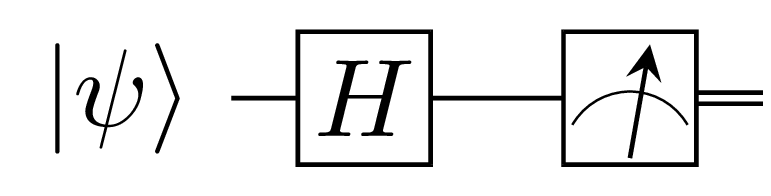Measurement of the $\sigma^{(x)}$ observable: we need to perform a basis change from the $\sigma^{(z)}$ basis to the $\sigma^{(x)}$ basis using $H$ then perform a standard measurement in the $\sigma^{(z)}$ basis. We will get eigenvectors in the $\sigma^{(z)}$ basis but the probabilities will correspond to measurements of the eigenvalues of $\sigma^{(x)}$.
• Code for performing the measurement
We prepare the state $\ket{\psi} = X\ket{0}$ and measure the $\sigma^{(x)}$ observable with respect to that state.
Measurement of the $\sigma^{(x)}$ observable: we prepare the state $\ket{\psi} = X\ket{0}$. We note that we obtain eigenvalue $+1$ with appromixately $0.5$ probablity and same for eigenvalue $-1$. It is easy to verify that this corresponds to theoretical predictions.
Also, note that measure() and custom_measure() provide the same results. In the first case, we use facilities provided by PennyLane. In the second case, we use the derived circuit.

Note that if we prepared the eigenvectors of $\sigma^{(x)}$ we will obtain eigenvalues with $100\%$ probability. That is if we prepare the $\ket{+} = H\ket{0}$ state, we will obtain eigenvalue $+1$ with $100\%$ probability.
Equivalently, if we prepare $\ket{-} = H\ket{1}$, we will obtain eigenvalue $-1$ with $100\%$ probability.

#### Multi-qubits measurement

In order to perform measurements on multiple qubits, we only need to perform a change of basis on each qubit individually as dictated by the form of the Hamiltonian.

Let us justify this: we will only consider the case of two qubits though the procedure can be proven for an arbitrary number of qubits.

Consider a generic 2-qubits Hamiltonian of the form $H = \sigma^{(m)} \otimes \sigma^{(n)}$, where $n, m \in \{i, x, y, z\}$. We would like to know how to find the probabilities corresponding to the eigenvalues of $H$.

First, we find the projectors:

\begin{align} H &= \sum_i \lambda^{(m)}_i P^{(m)}_i \otimes \sum_j \lambda^{(n)}_j P^{(n)}_j \\ &= \sum_{i,j} \lambda^{(m)}_i \cdot \lambda^{(n)}_j \left(P^{(m)}_i \otimes P^{(n)}_j\right) \\ &= \sum_r \lambda_r P_r \end{align}

Where we set $\lambda_r = \lambda^{(m)}_i \cdot \lambda^{(n)}_j$ and $P_r = P^{(m)}_i \otimes P^{(n)}_j$.

Then, we find the probability of measuring an arbitrary eigenvalue $\lambda_r$. Again, we only make the derivation for the 2-qubits case and make a general statement for the multiple-qubits case:

\begin{align} p(\lambda_r) &= \bra{\psi} P_r \ket{\psi} \\ &= \bra{\psi} (P_i^{(m)} \otimes P_j^{(n)}) \ket{\psi} \\ &= \bra{\psi} \left(\ket{m}\bra{m} \otimes \ket{n}\bra{n}\right) \ket{\psi} &P^{(\star)} = \ket{\star}\bra{\star} \\ &= \bra{\psi} \left((\overbrace{G_m\ket{0}}^{A}\overbrace{\bra{0}G_m^\dagger}^{B}) \otimes (\overbrace{G_n\ket{0}}^{C}\overbrace{\bra{0}G_n^\dagger}^{D}) \right) \ket{\psi} &\ket{\star}=G_\star\ket{0} \\ &= \bra{\psi} \left( (\overbrace{G_m}^{A'}\overbrace{\ket{0}}^{B'} \otimes \overbrace{G_n}^{C'}\overbrace{\ket{0}}^{D'}) (\overbrace{\bra{0}}^{A'}\overbrace{G_m^\dagger}^{B'} \otimes \overbrace{\bra{0}}^{C'}\overbrace{G_n^\dagger}^{D'}) \right) \ket{\psi} &(AB)\otimes(CD)=(A\otimes C)(B\otimes D) \\ &= \Big( \bra{\psi} (G_m \otimes G_n) (\ket{0} \otimes \ket{0})\Big)\Big((\bra{0} \otimes \bra{0}) (G_m^\dagger \otimes G_n^\dagger) \ket{\psi} \Big) &(A'B')\otimes(C'D')=(A'\otimes C')(B'\otimes D') \\ &= \lvert \bra{00} G_m^\dagger \otimes G_n^\dagger \ket{\psi} \rvert^2 \end{align}

The choice $\ket{\star} = G_\star \ket{0}$ is arbitrary. It could have been $\ket{\star}=G_\star\ket{1}$ and the result would still be similar.

The main point is that given a 2-qubits state $\ket{\psi}$ we just need to apply the gate $G_m^\dagger$ to the first qubit and the gate $G_n^\dagger$ to the second qubit then measure in the standard basis. If we had 3 qubits with $\sigma^{(o)}$ as the third observable acting on the third qubit, then we would apply gate $G_o^\dagger$ to the third qubit. And so on.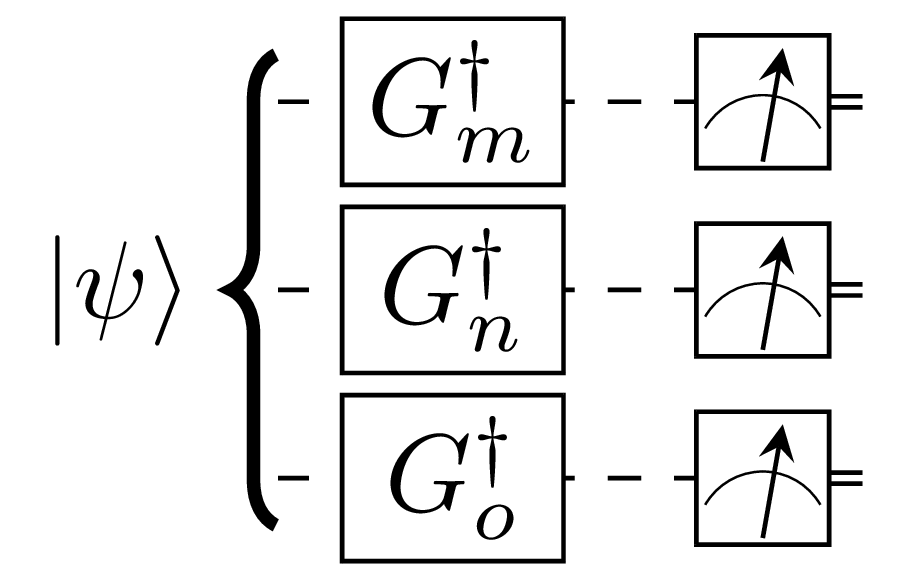Measurement of the $H= \sigma^{(m)} \otimes \sigma^{(n)} \otimes \sigma^{(o)}$ observable: we apply the corresponding changing of basis gates to each qubit indexed by the observable.
• Example 1: measurement of $H = \sigma^{(x)} \otimes \sigma^{(z)}$
As a first example, we will measure $H = \sigma^{(x)} \otimes \sigma^{(z)}$ with respect to one of its ground states.

So let’s start by finding the eigenvalues and eigenvectors of $H$. As the number of qubits increases, it gets difficult to do the calculations manually. That’s why we will use Numpy to do the calculation for us.

Eigenvalues and eigenvectors of $H = \sigma^{(x)} \otimes \sigma^{(z)}$: we find that $H$ has a degenerate ground state energy, that is there are two states with the same ground state energy $-1$.

We therefore see that $H$ has the following eigenvalues and eigenvectors:

1. Eigenvalue $-1$ has eigenvectors:
• $\dfrac{1}{\sqrt{2}} \begin{bmatrix} 1 & 0 & -1 & 0\end{bmatrix}^\intercal$
• $\dfrac{1}{\sqrt{2}} \begin{bmatrix} 0 & 1 & 0 & 1\end{bmatrix}^\intercal$
2. Eigenvalue $+1$ has eigenvectors:
• $\dfrac{1}{\sqrt{2}} \begin{bmatrix} 1 & 0 & 1 & 0\end{bmatrix}^\intercal$
• $\dfrac{1}{\sqrt{2}} \begin{bmatrix} 0 & -1 & 0 & 1\end{bmatrix}^\intercal$

Therefore, should we prepare the state $\ket{\psi} = \dfrac{1}{\sqrt{2}} \begin{bmatrix} 1 & 0 & -1 & 0\end{bmatrix}^\intercal = \dfrac{1}{\sqrt{2}}(\ket{00}-\ket{10})$, we should expect to measure eigenvalue $-1$ with probability $1$. The circuit that prepares that state and performs the measurement of $H$ against that state follows: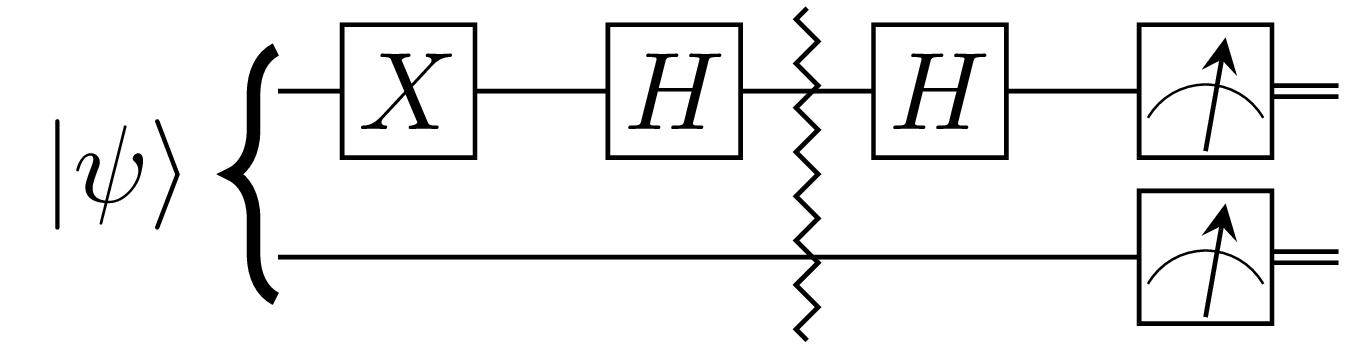Measurement of $H = \sigma^{(x)} \otimes \sigma^{(z)}$ against the state $\ket{\psi} = \dfrac{1}{\sqrt{2}}(\ket{00}-\ket{10})$: the gates before the zigzag lines correspond to the state preparation. The gates afterwards correspond to the change of basis before performing the measurement in the standard basis.
A simplification would result in cancellation of two $H$ gates acting on the first qubits. They are left for the purpose of clarity and completeness.

The code that follows implements the circuit above. The regular measure() function shows the implementation using PennyLane facilities. The custom_measure() does the implementation as per the figure above.

Measurement of $H$: Both measure() and custom_measure() should yield eigenvalue $-1$ with probability $1$.
Exercise
The reader is encouraged to find the circuits that prepare the remaining $3$ eigenvectors and verify that the corresponding eigenvalues are computed with the predicted probability of $100\%$.
• Example 2: measurement of $H = \sigma^{(z)} \otimes \sigma^{(i)}$
For our second example, we will measure $H = \sigma^{(z)} \otimes \sigma^{(i)}$ with respect to one of its ground states.

The eigenvalues and eigenvectors are calculated as before and are found to be:

1. Eigenvalue $-1$ has eigenvectors:
• $\begin{bmatrix} 0 & 0 & 1 & 0\end{bmatrix}^\intercal$
• $\begin{bmatrix} 0 & 0 & 0 & 1\end{bmatrix}^\intercal$
2. Eigenvalue $+1$ has eigenvectors:
• $\begin{bmatrix} 1 & 0 & 0 & 0\end{bmatrix}^\intercal$
• $\begin{bmatrix} 0 & 1 & 0 & 0\end{bmatrix}^\intercal$

We will prepare $\ket{\psi} = \begin{bmatrix} 0 & 0 & 0 & 1\end{bmatrix}^\intercal = \ket{11}$ and measure $H$ against that state.

The circuit that prepares $\ket{\psi}$ and measures $H$ against that state is in the figure that follows: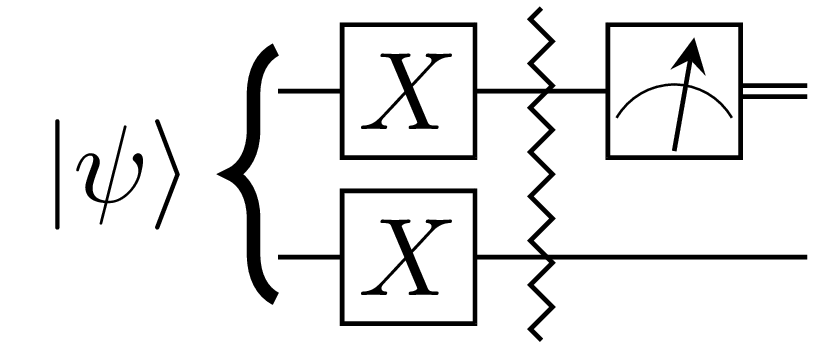Measurement of $H = \sigma^{(z)} \otimes \sigma^{(i)}$ against the state $\ket{\psi} = \ket{11}$: we need only measure the first qubit. This is equivalent to measuring both qubits in the standard basis.

The code that follows implements the figure above. Notice how we don’t tensor $\sigma^{(z)}$ with $\sigma^{(i)}$ in the code. We just perform a measurement on the first qubit.

Measurement of $H$: we only need to measure the first qubit when handed a Hamiltonian where there is a tensor with the identity.
Exercise
The reader is encouraged to find the circuits that prepare the remaining $3$ eigenvectors and verify that the corresponding eigenvalues are computed with the predicted probability of $100\%$.

### Expectation values

From equation $(\href{#mjx-eqn:2}{2})$ we note that measurements in quantum mechanics are inherently probabilistic in nature. That means we need to make multiple measurements in order to make coherent conclusions.

The fact that measurements are probablistic also means that we can calculate quantities such as moments of the probability distribution we get. Of particular interest we want to know the expectation value of the observale of interest.
In more words, given a state $\ket{\psi}$ and an observable $H$, we will generally want to know the expectation value ($i.e.$ average) of the eigenvalues ($i.e.$ energies since we have a Hamiltonian) with respect to that state.

In other words, we can’t really measure in one measuremement the energy of the system but we can only try to find the average. If the state against which we are making measurements happens to be an eigenvector of the Hamiltonian then after a sufficient number of measurements we will approach the true eigenvalue (that is the true energy of the system) with respect to that state.

So how do we find the average energy of a Hamiltonian given a state? From basic probability theory, the average is simply the sum of the measured energies times the probability of obtaining that specific energy.

\begin{align} \mathbb{E}(H) & = \sum_{i} \lambda_i p(\lambda_i) \\ & = \sum_{i} \lambda_i \bra{\psi} P_i \ket{\psi} \\ & = \bra{\psi} \left( \sum_{i} \lambda_i P_i \right) \ket{\psi} \\ & = \bra{\psi} H \ket{\psi} \end{align}

As a notational convenience, we will adopt:

$\braket{H} = \mathbb{E}(H) = \bra{\psi} H \ket{\psi} \tag{3}$

Using equation $(1’)$ for the expansion in the Hamiltonian eigenbasis, we obtain:

\begin{align} \braket{H} & = \bra{\psi} H \ket{\psi} \\ & = \bra{\psi} \left( \sum_{i} \lambda_i \ket{\lambda_i} \bra{\lambda_i} \right) \ket{\psi} \\ & = \sum_{i} \lambda_i \braket{\psi \\|\lambda_i} \braket{\lambda_i\\|\psi} \\ & = \sum_{i} \lambda_i \braket{\psi\\|\lambda_i} \braket{\psi\\|\lambda_i}^* \\ & = \sum_{i} \lambda_i \lvert\braket{\psi\\|\lambda_i}\rvert^{2} \end{align}

Therefore if we are given a state $\ket{\psi}$ and the eigendecomposition of $H$, we can find the expectation value using:

$\braket{H} = \sum_{i} \lambda_i \lvert\braket{\psi\\|\lambda_i}\rvert^{2} = \sum_{i} \lambda_i p(\lambda_i) \tag{3'}$

If the observable is of the form $H = \sum_i h_i H_i$ then the expectation value of $H$ is easily verified to be given by:

$\braket{H} = \sum_i h_i \braket{H_i} \tag{4}$

#### Example 1: expectation value of $H = \sigma^{(x)}$

We quickly confirm that if we prepare the ground state of $\sigma^{(x)}$, the expectation value will correspond to the ground state energy of $\sigma^{(x)}$ since we will obtain the ground state energy $-1$ with probability $1$

Measurement of $H = \sigma^{(x)}$: the circuit prepare the ground state of $H = \sigma^{(x)}$ therefore the expectation value will be the ground state energy.

#### Example 2: expectation value of $H = \dfrac{1}{\sqrt{2}}\left(\sigma^{(x)}+\sigma^{(z)}\right)$

A quick calculation shows that $H$ has the following eigendecomposition:

1. Eigenvalue $-1$ with eigenvector $\dfrac{1}{\sqrt{4+2\sqrt{2}}} \begin{bmatrix} 1-\sqrt{2} \\ 1\end{bmatrix}$
2. Eigenvalue $+1$ with eigenvector $\dfrac{1}{\sqrt{4+2\sqrt{2}}} \begin{bmatrix} 1+\sqrt{2} \\ 1\end{bmatrix}$

Unlike the previous examples, it is not clear how to prepare the ground state by inspection. So we can’t readily generate a circuit and compute the expectation value that would result in the ground state energy.

The ground state is generally unkown
If we knew the ground state, we would not need VQE because computing the ground state energy would simply amount to preparing the ground state and measuring the expectation value of the Hamiltonian with respect to the prepared state.

So we will prepare some generic state and measure the expectation value with respect to that state. The result is not important, it is how we achieve that result that’s important.

Note: PennyLane doesn’t exactly make it possible to iterate through the counts we get upon measurement so it is not easy for us to manually compute the expectation value according to equation $(\href{#mjx-eqn:3’}{3’})$. We will directly use their provided function for computing the expectation value and do a simple sanity check.

In the code that follows, we compute the expectation value according to equation $(\href{#mjx-eqn:4}{4})$ then ask PennyLane do the same calculation for us and compare the result.

The sanity check depends on the fact that PennyLane already has our observable $H$ as the $Hadamard$ observable.

Expectation value of $H = \dfrac{1}{\sqrt{2}}\left(\sigma^{(x)}+\sigma^{(z)}\right)$: we see a confirmation of equation $(\href{#mjx-eqn:4}{4})$ since both custom_expval and builtin_expval contain the same value.

#### Example 3: expectation value of $H = \sigma^{(x)} \otimes \sigma^{(z)} + \sigma^{(i)} \otimes \sigma^{(z)}$

It is easily and quickly verified that $H$ has the following eigendecomposition:

1. Eigenvalue $-2$ has eigenvector $\dfrac{1}{\sqrt{2}} \begin{bmatrix} 0 & 1 & 0 & 1\end{bmatrix}^\intercal$
2. Eigenvalue $0$ has two eigenvectors:
• $\dfrac{1}{\sqrt{2}} \begin{bmatrix} 1 & 0 & -1 & 0\end{bmatrix}^\intercal$
• $\dfrac{1}{\sqrt{2}} \begin{bmatrix} 0 & -1 & 0 & 1\end{bmatrix}^\intercal$
3. Eigenvalue $2$ has eigenvector $\dfrac{1}{\sqrt{2}} \begin{bmatrix} 1 & 0 & 1 & 0\end{bmatrix}^\intercal$

Therefore, if we compute the expectation value with respect to the state $\ket{\psi} = \dfrac{1}{\sqrt{2}} \left( \ket{01} + \ket{11} \right)$ we should get the eigenvalue $-2$. The code below confirms that.

Expectation value of $H = \sigma^{(x)} \otimes \sigma^{(z)} + \sigma^{(i)} \otimes \sigma^{(z)}$: since we prepared the ground state $\ket{\psi} = \ket{+}\ket{1}$ the expectation value yields the ground state energy $-2$.

### The variational method

From basic quantum mechanics we know that every system has a lowest energy, call it $\lambda_0$. We are generally interested in finding that energy.

The variational method allows to find an approximation of that energy. The idea is very simple: since $\lambda_0 \le \lambda_i, \forall i$, we have the following:

\begin{align} \braket{H} & = \sum_{i} \lambda_i \lvert\braket{\psi\\|\lambda_i}\rvert^{2} \\ & \ge \sum_{i} \lambda_0 \lvert\braket{\psi\\|\lambda_i}\rvert^{2} \\ & = \lambda_0 \sum_{i} \lvert\braket{\psi\\|\lambda_i}\rvert^{2} \\ & = \lambda_0 \sum_{i} p(\lambda_i) \\ & = \lambda_0 \end{align}

Where $\sum_{i} p(\lambda_i) = 1$ because probabilities must sum to $1$ for normalized states.

It follows then that:

$\braket{H} \ge \lambda_0 \tag{5}$

Equation $(\href{#mjx-eqn:4}{4})$ is the essence of the variational method. It tells us that we can always try to find some state that approximates the ground state. Our goal therefore is to keep constructing such some state and measure until we can’t find a state with a lowest energy because we can never find a state with lower energy than the ground state energy. Then we hope that the state found with lowest energy is sufficiently close to the true ground state energy.

### The variational algorithm

How then do we find the state $\ket{\psi}$ that approximates the ground state $\ket{\lambda_0}$? The trick is to parametrize $\ket{\psi}$ and then vary those parameters until a particular sequence of parameters leads to a state that appromiximates the ground state.

In other words we consider a state $\ket{\psi(\vec{\theta})} = U(\vec{\theta})\ket{00\dots0}$ where $\vec{\theta} = [\theta_{n-1},\theta_{n-2}, \cdots, \theta_0]$ are the parameters that we will vary until we appromixate the ground state. Whence the variational aspect of the algorithm.

#### Problem statement

Let us formaly state the variational problem. This is quite easy: find a sequence of parameters $\vec{\theta}$ such that $\mathcal{C}(\vec{\theta}) = \bra{\psi(\vec{\theta})}H\ket{\psi(\vec{\theta})} \approx \lambda_0$. The function $\mathcal{C}(\vec{\theta})$ is usually called the cost function or the objective function and our goal is to minimize it.

Mathematically:

$\underset{\vec{\theta}}{min} \: \mathcal{C}(\vec{\theta}) = \underset{\vec{\theta}}{min} \: \bra{\psi(\vec{\theta})}H\ket{\psi(\vec{\theta})} \ge \lambda_0$

That is our goal is to find parameters $\vec{\theta}$ that minimize $\mathcal{C}(\vec{\theta})$.

#### Problem solution

In general we will start with some arbitrary instance $\ket{\psi} = \ket{\psi(\vec{\theta})}$ where we fix $\vec{\theta}$ to some values (usually selected randomly). Then we will use an optimizer to find new parameters $\vec{\theta}^*$ such at $\mathcal{C}(\vec{\theta}^*) < \mathcal{C}(\vec{\theta})$.

The process will repeat itself until $\mathcal{C}(\vec{\theta}^*) \approx \lambda_0$ at which point the optimizer stops and reports the result.

The optimizer and local minima
The description above is a generalization because sometimes the optimizer can get stuck in a local minimum of $\mathcal{C}(\vec{\theta})$ and won't find a value close to $\lambda_0$ but we won't worry about that. Our hope though is that we end up with a value close to $\lambda_0$.

Each state $\ket{\psi(\vec{\theta})}$ where $\vec{\theta}$ is fixed is called an ansatz. We will use circuits that have arbitrary rotations about some axis to construct those ansätze. The circuits used to prepare arbitrary ansätze are called parametrized quantum circuits ($a.k.a.$ PQCs). Without loss of generality, we will use PQC and ansatz interchangeably.

#### The algorithm

While there will be slight variations in implementations, the flow of VQE is quite the same across all implementations. We present that flow as an algorithm:

Prepare:
$\quad cost(\vec{\theta}) = \bra{\psi(\vec{\theta})}H\ket{\psi(\vec{\theta})}$
$\quad optimizer = Optimizer()$

Initialize:
$\quad maxiter > 0$
$\quad iter = 0$
$\quad \vec{\theta} = rand()$
$\quad energy = cost(\vec{\theta})$

while $iter < maxiter$:
$\qquad \vec{\theta}, energy \gets optimizer(cost, \vec{\theta})$
$\qquad iter \gets iter + 1$

return $energy$

VQE algorithm: we prepare the cost function as a circuit that calculates the expectation value w.r.t. $\ket{\psi(\vec{\theta})}$, initialize the parameters $\vec{\theta}$ to some random values and let the optimizer take it from there for a maximum number of iterations after which we report the computed energy.

### Examples

Let us work through a couple of examples where we try to find their ground state energies. We have calculated those energies before, now we use VQE to find the same.

• Ansatz design:
For both examples, we rely on arbitrary state preparation circuits. This means that starting from fiduciary states $\ket{0}$ and $\ket{00}$, we create circuits that allow us to generate arbitrary single-qubit and two-qubits states.
The PQC for single-qubit systems is derived in single-qubit state preparation.
And a PQC for two-qubits systems is derived in two-qubits state preparation.

• Optimizer selection:
We chose the SPSA optimizer because it works out of the box without requiring additional knowledge beyond what we have already learned thus far. When we look at gradient descent, we will see we require the ability to find the gradient of the cost function and we haven’t learned how.

#### Example 1: ground state energy of $H = \dfrac{1}{\sqrt{2}}\left(\sigma^{(x)}+\sigma^{(z)}\right)$

We already know from calculating the expectation value of $H$ that it has ground state energy $-1$. We just couldn’t manually construct the ground state itself.

The code that follows implements VQE as per the algorithm above and it does find the ground state energy. We plot the optimization steps in the figure that follows the code so we can see the optimizer in action.

Ground state energy of $H = \dfrac{1}{\sqrt{2}}\left(\sigma^{(x)}+\sigma^{(z)}\right)$: while we may not get exactly $-1$, we will get comfortably close to it.

We can see how the optimizer gets closer to the ground state energy even though the initial energy estimation is not too far away from the true energy:

Optimization evolution every 10 steps: sometimes we will start close to the true ground state energy, other times not. But we clearly see the optimizer converging towards the true ground state energy.

The plot generated by the code above should help drive home the point of how VQE works: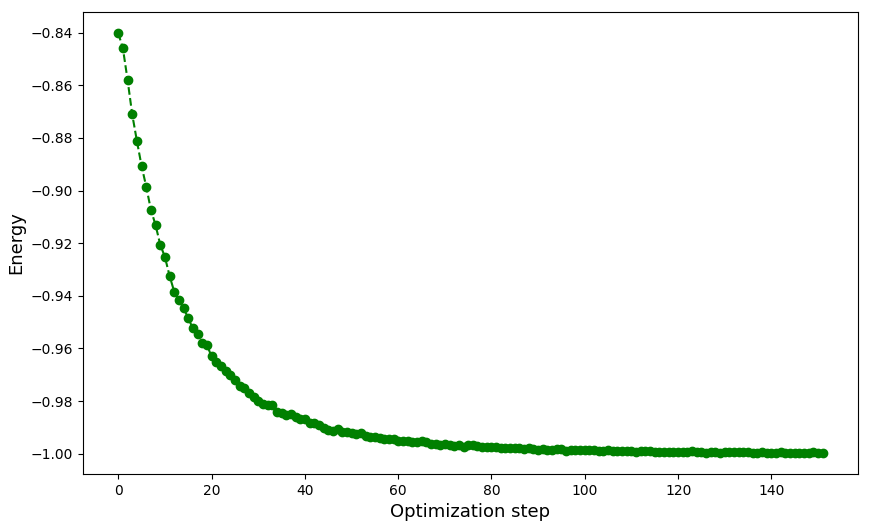VQE optimization landscape for $H = \dfrac{1}{\sqrt{2}}\left(\sigma^{(x)}+\sigma^{(z)}\right)$: we can clearly see the optimizer approaching the true ground state energy of our Hamiltonian $H$.

#### Example 2: ground state energy of $H = \sigma^{(x)} \otimes \sigma^{(z)} + \sigma^{(i)} \otimes \sigma^{(z)}$

The procedure is pretty much the same as with the Hadamard observable, except we will make use of equation $(\href{#mjx-eqn:4}{4})$ since we will calculate the ground state energies of $\sigma^{(x)} \otimes \sigma^{(z)}$ and $\sigma^{(i)} \otimes \sigma^{(z)}$ separately.

Without further ado, here is the code:

Ground state energy of $H = \sigma^{(x)} \otimes \sigma^{(z)} + \sigma^{(i)} \otimes \sigma^{(z)}$: we should expect to get an energy close to $-2$.

And here is a sample run on my machine:

Optimization evolution every 40 steps and final energy: while we didn't get exactly $-2$, we got pretty close to it.

### Next steps

The reader who just wanted to get the basics and play a little should free to stop here.

Even if the reader just wanted to get the basics, they are encouraged to read the final section on practical considerations so they understand the limitations of VQE, especially the measurement problem.

For the reader that wants to delve a little deeper, the sections that follow elaborate on ways ansätze are designed, what choices of optimizers we have, and a few ways we have to reduce the number of measurements.

## Ansatz design

Designing an ansatz is certainly no easy task. Building up on the work already done, ansätze can be classified into fixed structure or adaptive structure ansätze, see (Tilly et al., 2022).

The software engineer will generally experiment with various ansätze for a specific problem via educated guessing.
And when appropriate build upon existing ansätze.

Fixed structure ansätze are those where the circuit structure doesn’t change between optimization phases. In adaptive strucutre ansätze, the circuit structure changes as the circuit structure is adapted to the problem being solved.

Moreover, even in fixed structure ansätze, we will try to restrict ourselves to ansätze that a software engineer can tackle.

In fixed structure ansätze, I do the following classification:

1. Physics inspired ansätze.
2. Hardware inspired ansätze.
3. Mathematics inspired ansätze.

### Physics inspired ansätze

In physics inspired ansätze, we try to use information about the problem and decide how best to tailor the ansatz such that we can efficiently search the Hilbert space.

There are two ansätze that have featured prominently with applications in quantum chemistry and condensed matter physics.

Since we are software engineers, it is not worth our while to study these ansätze in detail, it is sufficient to know they exist.

#### Unitary coupled cluster (UCC) ansatz

This is the ansatz that was used in the original work on VQE (Peruzzo et al., 2014). It comes from coupled cluster theory for studying many-body systems and has found many uses in ab initio quantum chemistry.

#### Hamiltonian variational ansatz (HVA)

The Hamiltonian variational ansatz builds upon the Hamiltonian to be studied. The circuit is built by taking the exponential of commuting terms in the Hamiltonian. That procedure gives us unitaries that can be decomposed into gates.

The paper that proposed this ansatz, (Wecker et al., 2015) shows that the ansatz doesn’t perform very well in quantum chemistry applications but does well on the Hubbard model, a condensed matter physics problem.

Since condensed matter physics is one of the fields where we expect quantum computing to help, if faced with a problem from condensed matter physics, HVA is a good ansatz to try out.

### Hardware inspired ansätze

The basic idea of hardware inspired ansätze is that we create a circuit that closely matches the structure of the hardware, most importantly the native gateset, and if possible the connectivity of the device.

This type of ansätze came from (Kandala et al., 2017) and the original is called hardware-efficient ansatz (HEA). There are many variants of it now but this one is sufficient to get start.

#### Hardware-efficient ansatz (HEA)

The fundemental structure of the hardware-efficient ansatz is to start with a layer of rotations gates acting individualy on each qubit as in the figure that follows: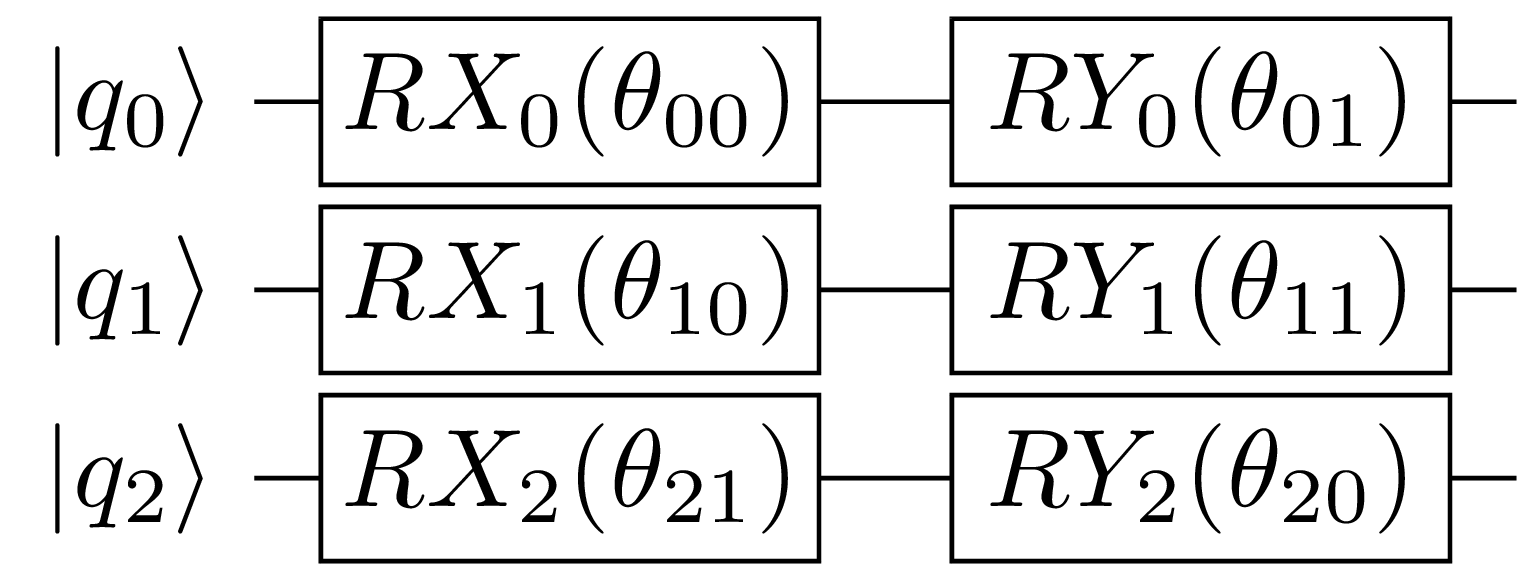Possible rotation layer of HEA: we have chosen $RX$ and $RY$ as our rotation gates.

Then we follow that initialization step with a layer of entangling gates. Then an additional layer of rotations. Then entanglers, etc. We will generally want the entangling layer to reflect the topology of the device so we can avoid introducing additional $SWAP$ gates to account for qubits that are not connected directly on the device. This layer will look something like what follows in the figure below: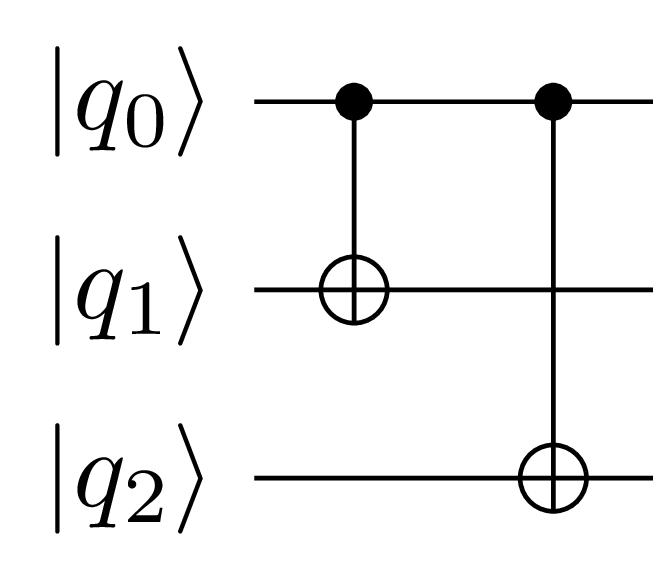Possible entangling layer of HEA: we have chosen $CNOT$ gates as our entanglers.

The parametrized quantum circuit for HEA will have a general structure as per the formula that follows:

\begin{align} \ket{\psi(\vec{\theta})} &= \prod_{q=1}^{N} \left[ U^{q,d-1}(\vec{\theta}) \right] \times U_{ENT} \\ &\times \prod_{q=1}^{N} \left[ U^{q,d-2}(\vec{\theta}) \right] \times U_{ENT} \\ &\times \cdots \times \\ &\times \prod_{q=1}^{N} \left[ U^{q,0}(\vec{\theta}) \right] \ket{00\dots0} \end{align}

Where $q$ is the qubit index up to $N$ qubits and $d$ is number of layers since we are allowed to repeat the various rotation and entangling layers. $U^{q,l}(\vec{\theta})$ is the layer of rotations acting on $q$ qubits in the $d^{th}$ layer. Note that if a layer in a layer has $M$ rotation gates per qubits acting on $N$ qubits, we will need $M \times N$ parameters per layer.

Exercise
The reader is encouraged to use HEA for the two-qubits example(s) we have encounted thus far and explore with changing the number of layers.

### Mathematics inspired ansätze

In this class of ansätze, we don’t look at the make of the hardware nor do we take into consideration the structure of the problem.

The premise is this: how do we prepare arbitrary quantum states? There are two ways:

1. State preparation: start from a know state and ask what circuit would allow us to explore the Hilbert space starting from that state.
2. Gate synthesis: given an arbitrary starting state, what circuit would allow us to explore the entire Hilbert space?

We have already encountered ansätze based on state preparation so there should be no suprise there. The gate synthesis problem on the other hand is based on create a unitary that prepares an arbitrary quantum state then decomposing that unitary into a given gateset (that of the device we will run on).

Both approaches fall into the area of quantum compiling and this subsection cannot do it justice. We will therefore only broach the subject and point the reader to a couple of resources.

#### State preparation ansatz

We learned how to prepare states starting from $\ket{00\dots0}$ in the previous sections and derived circuits for one and two qubits circuits.

But we also learned that the circuits derived are inefficient with regard to the number of $CNOT$ gates. This is important because $CNOT$ gates are known to be very noisy so they reduce the quality of our ansatz.

For two qubits, for instance, it is known that we would not need more than a single $CNOT$ gate using the Schmidt decomposition.

The method can be generalized to multiple qubits and is elaborate upon in (Murta et al., 2023).

Exercise
The reader is encouraged to read section VII of the paper (Murta et al., 2023) and implement the procedure elaborate upon therein.

#### Gate synthesis ansatz

In the gate synthesis ansatz, we build a circuit that is capable of preparing any quantum state irrespective of the starting state.

This may not be as efficient as the Schmidt decomposition but it is still a valid procedure to build ourselves ansätze. For instance, the gate synthesis for two qubits will produce a circuit with 3 $CNOT$ gates.

A circuit for two qubits gate synthesis is presented in (Shende et al., 2004).

Exercise
The reader is encouraged to implement a generic circuit for single and two qubits gate synthesis and compare the results and optimizations with other types of ansätze already studied, mainly HEA and state preparation based ansätze.

## Optimizer selection

Optimizers are generally classified into two groups:

1. Gradient-based optimizers: these require computing or approximating the gradient of the objective function.
2. Gradient-free optimizers: no need to compute or approximate the gradient of the objective function.

The choice of an optimizer will generally depend on how fast it converges to the solution, how many measurements it needs, and the quality of the solution obtained.

If exploring optimizers is not your goal, SPSA is good starting point (Bonet-Monroig et al., 2023).

Gradient-based methods require computing the gradient directly or approximating it in some form.

For optimization by direct gradient computation, we discuss gradient-descent. For optimization for gradient approximation, we discuss SPSA.

The idea of gradient-descent is very simple: given a cost function, we calculate its gradient then use the it to find the new set of parameters. The update rule is given by the following equation:

\begin{align} \vec{\theta_{k+1}} = \vec{\theta_k} - \eta \nabla C(\vec{\theta_k}) \end{align}

Where $\eta$ is the learning rate or step size. It tells us how big a step to take towards finding the minimum.

At step $n = 0$, we might start with random parameters, calculate the gradient with respect to those parameters then compute the next set of parameters $n = 1$.

The reader might wander how exactly the gradient of the expectation value is calculated; that is finding:

\begin{align} \nabla C(\vec{\theta_k}) = \nabla(\bra{\psi(\vec{\theta})} H \ket{\psi(\vec{\theta})}) = \bra{0\dots00} \nabla U^{\dagger}(\vec{\theta}) H \nabla U(\vec{\theta}) \ket{00\dots0} \end{align}

This problem is solved using a method called parameter-shift rule, introduced in (Mitarai et al., 2018). We won’t elaborate on the details, the interested reader can get a quick introduction from PennyLane.

Gradient-descent has many variants, so it is really interesting to play with them using different Hamiltonians and different PQCs. The book Algorithms for Optimization (Kochenderfer & Wheeler, 2019) has a plethora of such algorithms for the interested reader to play with.

#### Simultaneous perturbation stochastic approximation (SPSA)

The idea with SPSA is to replace direct gradient evaluation with gradient approximation, that is replacing $\nabla C(\vec{\theta_k})$ with an estimator $g_{k}(\vec{\theta_k})$. The new update rule is given by:

\begin{align} \vec{\theta_{k+1}} = \vec{\theta_k} - a_k g_{k}(\vec{\theta_k}) \end{align}

Where $a_k$ is a positive number and $g_{k}(\vec{\theta_k})$ is obviously the gradient estimator.

The estimator $g_{k}(\vec{\theta_k})$, being a vector, will have its $i^{th}$ component computed as follows:

\begin{align} (g_{k}(\vec{\theta_k}))_i = \frac{C(\theta_k + c_k \Delta_k) - C(\theta_k - c_k \Delta_k)}{2c_k(\Delta_{k})_i} \end{align}

Where $c_k$ is a user-supplied positive number and $\Delta_k = \begin{bmatrix} \Delta_{k_1} & \Delta_{k_2} & \cdots & \Delta_{k_p} \end{bmatrix}^\intercal$ is a random perturbation vector with $p$ entries corresponding to the number of parameters in the PQC.

The advantage of SPSA over gradient descent is that it evaluates the objective function only twice compared to GD that evaluates it $p$ times.

Exercise
The reader is encouraged to run GD and SPSA on some problems and compare their convergence rates. The PennyLane tutorial on SPSA is a good starting point.

In gradient-free methods, not only we do not compute the gradient directly, we don’t even try to approximate it.

There are many such algorithms such as Nelder-Mead, Powell, Quantum analytic descent, etc.

While interesting in their own, I won’t try to even offer much description of them but the reader is encouraged to also experiment with them if they are so inclined.

## Measurement reduction

Quantum computers are a precious resource so we would like to make as few measurements as possible so we can solve a greater number of problems.

Let us recall that a Hamiltonian will be a linear combination of Pauli terms (tensored Pauli matrices): $H = \sum_i h_i H_i$.

There are two easy ways we can see in trying to reduce the number of measurements:

1. Measure commuting observables together.
2. Allocate more shots to Pauli terms with higher coefficients.

There are some other strategies that have been proposed but those two above are quite easy to understand so we will describe them.

### Grouping qubit-wise commutative Pauli terms

It is know that observables that do not commute cannot be measured simultaneously due to the Heisenberg uncertainty principle.

Conversely, if two observables commute they can be measured simultaneously.

Qubit-wise commutativity simply means that in a Pauli term if each Pauli matrix acting on qubit $i$ commutes with a Pauli matrix in another Pauli term then those two Pauli terms commute with each other.
This will be the case when the Pauli matrix in both terms is either itself or the identity.

For instance, in our two-qubits Hamiltonian $H = \sigma^{(x)} \otimes \sigma^{(z)} + \sigma^{(i)} \otimes \sigma^{(z)}$ both terms $H_1 = \sigma^{(x)} \otimes \sigma^{(z)}$ and $H_2 = \sigma^{(i)} \otimes \sigma^{(z)}$ commute qubit-wise because $\sigma^{(x)}$ commutes with $\sigma^{(i)}$ and $\sigma^{(z)}$ commutes with itself. That means that both terms of $H$ can be measured simultaneously.

If instead we had $H = \sigma^{(x)} \otimes \sigma^{(z)} + \sigma^{(z)} \otimes \sigma^{(z)}$ then we couldn’t measure both Pauli terms simultaneously because they are not qubit-wise commuting.

The essence of grouping is to find Pauli terms in a Hamiltonian that commute and measure them together therefore reducing the number of measurements needed to calculate the expectation value of the entire Hamiltonian.

### Weight distribution of measurements

We recall that the expectation value of $H = \sum_i h_i H_i$ is given by $\braket{H} = \sum_i h_i \braket{H_i}$. It follows that terms with higher coefficients will contribute more to the final expectation value than those with lower coefficients.

So the basic idea is to weight each Pauli term according to its coefficient then distribute shots according to the weight of each Pauli term.

In some cases, if some terms have very little weights, they might be dropped entirely without affecting much the final result.

## Practical considerations

There are a couple of challenges to consider when dealing with VQE:

1. The number of measurements.
2. Barren plateaus during training.

### The measurement problem

Compared to training neural networks, VQE sometimes require that we keep the optimization loop going until we have enough samples to calculate the expectation value up to a required precision.

This has proven challenging because it requires a huge number of measurements to achieve the need precision. (Gonthier et al., 2022) show that for quantum chemistry applications on relatively simple molecules, a single energy evaluation takes days!

This is a major limitation of VQE that’s a subject of current research.

### Barren plateaus problem

In order to do optimization, we saw that using gradient-based optimization, we need to evaluate the gradient of the cost function.

What (McClean et al., 2018) showed was that for a large class of parametrized quantum circuits the gradient will become zero during optimization and thus the optimization will not progress.

This is the trainability problem of VQE. What’s worse, the more expressive a PQC (meaning the more it can explore the Hilbert space), the more prone it is to the barren plateau problem.

So it is important to look for PQCs that are robust against barren plateaus.

## Conclusion

We introduced the variational quantum eigensolver(VQE) by doing thorough derivations of the basic components of how it works and wrote code that validate the algorithm.

The interested reader is given pointers in refining the different components of the algorithm so they can tackle more advanced applications.

We finally touched upon some practical considerations that highlight the limitations of VQE and where research is headed so as to make VQE of practical use.

## Derivations

Some derivations were not necessary to follow the main material but nonetheless are useful to know for completeness sake. This section contains those derivations for interested readers.

### Eigenvalues and eigenvectors of $\sigma^{(z)}$

#### Eigenvalues

\begin{align} \det\begin{vmatrix} \sigma^{(z)} - \lambda \sigma^{(i)} \end{vmatrix} &= 0 \\ \implies \det\begin{vmatrix} \begin{bmatrix} 1-\lambda & 0 \\ 0 & -1-\lambda \end{bmatrix} \end{vmatrix} &= 0 \\ \implies (1-\lambda)(-1-\lambda) &= 0 \\ \implies \lambda &= \pm 1 \end{align}

The eigenvalues of $\sigma^{(z)}$ are $\lambda_0 = +1$ and $\lambda_1 = -1$.

#### Eigenvectors

• Eigenvector corresponding to eigenvalue $\lambda_0 = +1$

\begin{align} \sigma^{(z)} \ket{\lambda_+} &= +1 \ket{\lambda_+} \\ \implies \begin{bmatrix} 1 & 0 \\ 0 & -1 \end{bmatrix} \begin{bmatrix} c_0 \\ c_1 \end{bmatrix} &= \begin{bmatrix} c_0 \\ c_1 \end{bmatrix} \\ \implies \begin{bmatrix} c_0 \\ -c_1 \end{bmatrix} &= \begin{bmatrix} c_0 \\ c_1 \end{bmatrix} \\ \implies \begin{cases} c_0 &= c_0 \\ -c_1 &= c_1 \end{cases} \end{align}

In the last step, $c_0 = c_0$ tells us nothing useful. But $-c_1 = c_1$ tells us that $c_1 = 0$. It follows then that $\ket{\lambda_+} = \begin{bmatrix} c_0 \\ 0 \end{bmatrix}$.

Using the normalization condition, we find that $\braket{\lambda_+|\lambda_+}=1$ implies $|c_0|^2=1$ therefore $c_0 = 1$.

Thus $\ket{\lambda_+} = \begin{bmatrix} 1 \\ 0 \end{bmatrix}$. This eigenvector is also written as $\ket{0} = \ket{\lambda_+}$.

• Eigenvector corresponding to eigenvalue $\lambda_1 = -1$
Repeating the same calculations as above:

\begin{align} \sigma^{(z)} \ket{\lambda_-} &= -1 \ket{\lambda_-} \\ \implies \begin{bmatrix} 1 & 0 \\ 0 & -1 \end{bmatrix} \begin{bmatrix} c_0 \\ c_1 \end{bmatrix} &= \begin{bmatrix} -c_0 \\ -c_1 \end{bmatrix} \\ \implies \begin{bmatrix} c_0 \\ -c_1 \end{bmatrix} &= \begin{bmatrix} -c_0 \\ -c_1 \end{bmatrix} \\ \implies \begin{cases} c_0 &= -c_0 \\ c_1 &= c_1 \end{cases} \end{align}

Following the same reasoning that we used to calculate $\ket{\lambda_+}$, we find that $c_0 = 0$ and $c_1=1$.

Thus $\ket{\lambda_-} = \begin{bmatrix} 0 \\ 1 \end{bmatrix}$. This eigenvector is also written as $\ket{1} = \ket{\lambda_-}$.

### Eigenvalues and eigenvectors of $\sigma^{(y)}$

#### Eigenvalues

\begin{align} \det\begin{vmatrix} \sigma^{(y)} - \lambda \sigma^{(i)} \end{vmatrix} &= 0 \\ \implies \det\begin{vmatrix} \begin{bmatrix} -\lambda & -i \\ i & -\lambda \end{bmatrix} \end{vmatrix} &= 0 \\ \implies \lambda^{2}-1 &= 0 \\ \implies \lambda &= \pm 1 \end{align}

The eigenvalues of $\sigma^{(y)}$ are $\lambda_+ = +1$ and $\lambda_- = -1$.

#### Eigenvectors

• Eigenvector corresponding to eigenvalue $\lambda_+ = +1$

\begin{align} \sigma^{(y)} \ket{\lambda_+} &= +1 \ket{\lambda_+} \\ \implies \begin{bmatrix} 0 & -i \\ i & 0 \end{bmatrix} \begin{bmatrix} c_0 \\ c_1 \end{bmatrix} &= \begin{bmatrix} c_0 \\ c_1 \end{bmatrix} \\ \implies \begin{bmatrix} -i c_1 \\ i c_0 \end{bmatrix} &= \begin{bmatrix} c_0 \\ c_1 \end{bmatrix} \\ \implies \begin{cases} -i c_1 &= c_0 \\ i c_0 &= c_1 \end{cases} \end{align}

Using $c_1 = i c_0$, we transform $\ket{\lambda_+}$ as follows: $\ket{\lambda_+} = \begin{bmatrix}c_0 \\ i c_0\end{bmatrix}$

Using the normalization condition, we find that $\braket{\lambda_+|\lambda_+}=1$ implies $2|c_0|^2=1$ from which it follows that $c_0 = \dfrac{1}{\sqrt{2}}$. Consequently $c_1 = \dfrac{i}{\sqrt{2}}$.

Thus $\ket{\lambda_+} = \dfrac{1}{\sqrt{2}} \begin{bmatrix} 1 \\ i \end{bmatrix}$. This eigenvector is also written as $\ket{+i} = \ket{\lambda_+}$. Expressed in the $\sigma^{(z)}$ basis, $\ket{+i} = \dfrac{1}{\sqrt{2}}(\ket{0} + i\ket{1})$.

• Eigenvector corresponding to eigenvalue $\lambda_- = -1$

\begin{align} \sigma^{(y)} \ket{\lambda_-} &= -1 \ket{\lambda_-} \\ \implies \begin{bmatrix} 0 & -i \\ i & 0 \end{bmatrix} \begin{bmatrix} c_0 \\ c_1 \end{bmatrix} &= \begin{bmatrix} -c_0 \\ -c_1 \end{bmatrix} \\ \implies \begin{bmatrix} -i c_1 \\ i c_0 \end{bmatrix} &= \begin{bmatrix} -c_0 \\ -c_1 \end{bmatrix} \\ \implies \begin{cases} i c_1 &= c_0 \\ i c_0 &= -c_1 \end{cases} \end{align}

Using $c_0 = i c_1$, we transform $\ket{\lambda_-}$ as follows: $\ket{\lambda_-} = \begin{bmatrix}ic_1 \\ c_1\end{bmatrix}$

Using the normalization condition, we find that $\braket{\lambda_-|\lambda_-}=1$ implies $2|c_1|^2=1$ from which it follows that $c_1 = \dfrac{1}{\sqrt{2}}$. Consequently $c_1 = -\dfrac{i}{\sqrt{2}}$.

Thus $\ket{\lambda_-} = \dfrac{1}{\sqrt{2}} \begin{bmatrix} 1 \\ -i \end{bmatrix}$. This eigenvector is also written as $\ket{-i} = \ket{\lambda_-}$. Expressed in the $\sigma^{(z)}$ basis, $\ket{-i} = \dfrac{1}{\sqrt{2}}(\ket{0} - i\ket{1})$.

### Eigenvalues and eigenvectors of $\sigma^{(x)}$

#### Eigenvalues

\begin{align} \det\begin{vmatrix} \sigma^{(x)} - \lambda \sigma^{(i)} \end{vmatrix} &= 0 \\ \implies \det\begin{vmatrix} \begin{bmatrix} -\lambda & 1 \\ 1 & -\lambda \end{bmatrix} \end{vmatrix} &= 0 \\ \implies \lambda^{2}-1 &= 0 \\ \implies \lambda &= \pm 1 \end{align}

The eigenvalues of $\sigma^{(x)}$ are $\lambda_+ = +1$ and $\lambda_- = -1$.

#### Eigenvectors

• Eigenvector corresponding to eigenvalue $\lambda_+ = +1$

\begin{align} \sigma^{(x)} \ket{\lambda_+} &= +1 \ket{\lambda_+} \\ \implies \begin{bmatrix} 0 & 1 \\ 1 & 0 \end{bmatrix} \begin{bmatrix} c_0 \\ c_1 \end{bmatrix} &= \begin{bmatrix} c_0 \\ c_1 \end{bmatrix} \\ \implies \begin{bmatrix} c_1 \\ c_0 \end{bmatrix} &= \begin{bmatrix} c_0 \\ c_1 \end{bmatrix} \\ \implies \begin{cases} c_1 &= c_0 \\ c_0 &= c_1 \end{cases} \end{align}

Using $c_1 = c_0$, we transform $\ket{\lambda_+}$ as follows: $\ket{\lambda_+} = \begin{bmatrix}c_0 \\ c_0\end{bmatrix}$

Using the normalization condition, we find that $\braket{\lambda_+|\lambda_+}=1$ implies $2|c_0|^2=1$ from which it follows that $c_0 = \dfrac{1}{\sqrt{2}}$. Consequently $c_1 = \dfrac{1}{\sqrt{2}}$.

Thus $\ket{\lambda_+} = \dfrac{1}{\sqrt{2}} \begin{bmatrix} 1 \\ 1 \end{bmatrix}$. This eigenvector is also written as $\ket{+} = \ket{\lambda_+}$. Expressed in the $\sigma^{(z)}$ basis, $\ket{+} = \dfrac{1}{\sqrt{2}}(\ket{0} + \ket{1})$.

• Eigenvector corresponding to eigenvalue $\lambda_- = -1$

\begin{align} \sigma^{(x)} \ket{\lambda_-} &= -1 \ket{\lambda_-} \\ \implies \begin{bmatrix} 0 & 1 \\ 1 & 0 \end{bmatrix} \begin{bmatrix} c_0 \\ c_1 \end{bmatrix} &= \begin{bmatrix} -c_0 \\ -c_1 \end{bmatrix} \\ \implies \begin{bmatrix} c_1 \\ c_0 \end{bmatrix} &= \begin{bmatrix} -c_0 \\ -c_1 \end{bmatrix} \\ \implies \begin{cases} c_1 &= -c_0 \\ c_0 &= -c_1 \end{cases} \end{align}

Using $c_0 = -c_1$, we transform $\ket{\lambda_-}$ as follows: $\ket{\lambda_-} = \begin{bmatrix}c_0 \\ -c_0\end{bmatrix}$

Using the normalization condition, we find that $\braket{\lambda_-|\lambda_-}=1$ implies $2|c_0|^2=1$ from which it follows that $c_0 = \dfrac{1}{\sqrt{2}}$. Consequently $c_1 = -\dfrac{1}{\sqrt{2}}$.

Thus $\ket{\lambda_-} = \dfrac{1}{\sqrt{2}} \begin{bmatrix} 1 \\ -1\end{bmatrix}$. This eigenvector is also written as $\ket{-} = \ket{\lambda_-}$. Expressed in the $\sigma^{(z)}$ basis, $\ket{-} = \dfrac{1}{\sqrt{2}}(\ket{0} - \ket{1})$.

### Parametrized quantum circuits via state preparation

We can use state preparation circuits as a starting point for the design of parametrized quantum circuits.

We do derivations for single-qubit and two-qubits states though the procedure can be extented to multiple qubits.

Note: except for the single-qubit case, any circuit of more than one qubit obtained by the procedure below will be inefficient depth-wise and CNOT count wise.

#### Single-qubit state preparation

A single qubit has the trigonometric parametrization:

\begin{align} \ket{\psi} = \cos\dfrac{\theta}{2} \ket{0} + {\rm e}^{i\phi} \sin\dfrac{\theta}{2} \ket{1} \end{align}

Our task then is to design a circuit that would prepare such a state starting from the state $\ket{0}$.

We begin by noting that $RY(\theta)\ket{0} = \cos\dfrac{\theta}{2} \ket{0} + \sin\dfrac{\theta}{2} \ket{1}$. We also know that application of the phase shift gate confers a phase to a qubit in the $\ket{1}$ state but does nothing to the $\ket{0}$ state.

So it follows that $P(\phi)RY(\theta)\ket{0} = \cos\dfrac{\theta}{2} \ket{0} + {\rm e}^{i\phi} \sin\dfrac{\theta}{2} \ket{1}$.

And thus we have our circuit: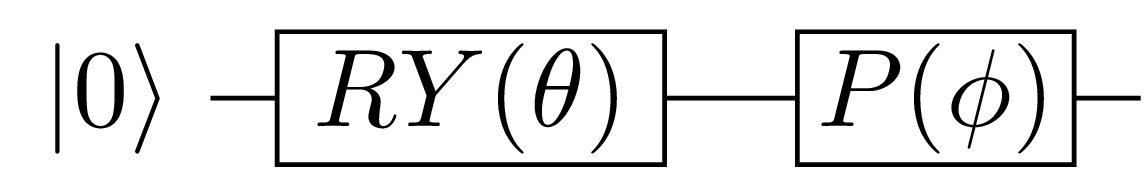Preparation of an arbitrary single qubit state: we apply a rotation about Y then a phase shift gate.

#### Two-qubits state preparation

A two-qubits state has the trigonometric parametrization:

\begin{align} \ket{\psi} &= \cos\dfrac{\theta_1}{2} \ket{00} \\ &+ {\rm e}^{i\phi_1} \sin\dfrac{\theta_1}{2} \cos\dfrac{\theta_2}{2} \ket{01} \\ &+ {\rm e}^{i\phi_2} \sin\dfrac{\theta_1}{2} \sin\dfrac{\theta_2}{2} \cos\dfrac{\theta_3}{2} \ket{10} \\ &+ {\rm e}^{i\phi_3} \sin\dfrac{\theta_1}{2} \sin\dfrac{\theta_2}{2} \sin\dfrac{\theta_3}{2} \ket{11} \\ \end{align}

We design the circuit by following the exact same steps as for the single qubit case, starting from the $\ket{00}$ state.

1. Apply $RY(\theta_1)$ to qubit $1$:

\begin{align} \ket{\psi_1} &= RY_{1}(\theta_1) \ket{00} \\ &= \cos\dfrac{\theta_1}{2} \ket{00} + \sin\dfrac{\theta_1}{2} \ket{01} \end{align}
2. Apply $P(\phi_1)$ to qubit $1$:

\begin{align} \ket{\psi_2} &= P_{1}(\phi_1) \ket{\psi_1} \\ &= \cos\dfrac{\theta_1}{2} \ket{00} + {\rm e}^{i\phi_1} \sin\dfrac{\theta_1}{2} \ket{01} \end{align}
3. Apply controlled-$RY(\phi_{2})$ to qubit $0$ if qubit $1$ is set:

\begin{align} \ket{\psi_3} &= CRY^{(1)}_{1\to 0}(\theta_2) \ket{\psi_2} \\ &= \cos\dfrac{\theta_1}{2} \ket{00} \\ &+ {\rm e}^{i\phi_1} \sin\dfrac{\theta_1}{2} \cos\dfrac{\theta_2}{2} \ket{01} \\ &+ \underbrace{ {\rm e}^{i\phi_1} }_{\text{wrong phase}} \sin\dfrac{\theta_1}{2} \sin\dfrac{\theta_2}{2} \ket{11} \end{align}

Comparing with the original state $\ket{\psi}$ we are trying to construct, it clear that the term ${\rm e}^{i\phi_1} \sin\dfrac{\theta_1}{2} \sin\dfrac{\theta_2}{2} \ket{11}$ in $\ket{\psi_3}$ has the wrong phase; it should be ${\rm e}^{i\phi_3}$ and not ${\rm e}^{i\phi_1}$. We do the correction in the next step.

4. Apply controlled-$P(\phi_3-\phi_1)$ to qubit $1$ if qubit $0$ is set:

\begin{align} \ket{\psi_4} &= CP^{(1)}_{0\to 1}(\phi_3 - \phi_1) \ket{\psi_3} \\ &= \cos\dfrac{\theta_1}{2} \ket{00} \\ &+ {\rm e}^{i\phi_1} \sin\dfrac{\theta_1}{2} \cos\dfrac{\theta_2}{2} \ket{01} \\ &+ {\rm e}^{i\phi_3} \sin\dfrac{\theta_1}{2} \sin\dfrac{\theta_2}{2} \ket{11} \end{align}
5. Apply controlled-$RY(\theta_3)$ to qubit $1$ if qubit $0$ is set:

\begin{align} \ket{\psi_5} &= CRY^{(1)}_{0\to 1}(\theta_3) \ket{\psi_4} \\ &= \cos\dfrac{\theta_1}{2} \ket{00} \\ &+ {\rm e}^{i\phi_1} \sin\dfrac{\theta_1}{2} \cos\dfrac{\theta_2}{2} \ket{01} \\ &+ \underbrace{ {\rm e}^{i\phi_3} }_{\text{wrong phase}} \sin\dfrac{\theta_1}{2} \sin\dfrac{\theta_2}{2} \cos\dfrac{\theta_3}{2} \ket{10} \\ &+ {\rm e}^{i\phi_3} \sin\dfrac{\theta_1}{2} \sin\dfrac{\theta_2}{2} \sin\dfrac{\theta_3}{2} \ket{11} \\ \end{align}

Again, we see that there is a term with the wrong phase, ${\rm e}^{i\phi_3} \sin\dfrac{\theta_1}{2} \sin\dfrac{\theta_2}{2} \cos\dfrac{\theta_3}{2} \ket{10}$. It should be ${\rm e}^{i\phi_2}$ and not ${\rm e}^{i\phi_3}$. We correct the phase in the next step.

6. Apply controlled-$P(\phi_2 - \phi_3)$ to qubit $0$ if qubit $1$ is not set:

\begin{align} \ket{\psi_6} &= CP^{(0)}_{0\to 1}(\phi_2 - \phi_2) \ket{\psi_5} \\ &= \cos\dfrac{\theta_1}{2} \ket{00} \\ &+ {\rm e}^{i\phi_1} \sin\dfrac{\theta_1}{2} \cos\dfrac{\theta_2}{2} \ket{01} \\ &+ {\rm e}^{i\phi_2} \sin\dfrac{\theta_1}{2} \sin\dfrac{\theta_2}{2} \cos\dfrac{\theta_3}{2} \ket{10} \\ &+ {\rm e}^{i\phi_3} \sin\dfrac{\theta_1}{2} \sin\dfrac{\theta_2}{2} \sin\dfrac{\theta_3}{2} \ket{11} \end{align}

Notice that the term $\cos\dfrac{\theta_1}{2} \ket{00}$ doesn’t acquire any phase. This is because, remember, the target qubit is in the $\ket{0}$ state.

And we have recovered the original state $\ket{\psi}$. The circuit corresponding to the gates sequences is presented below: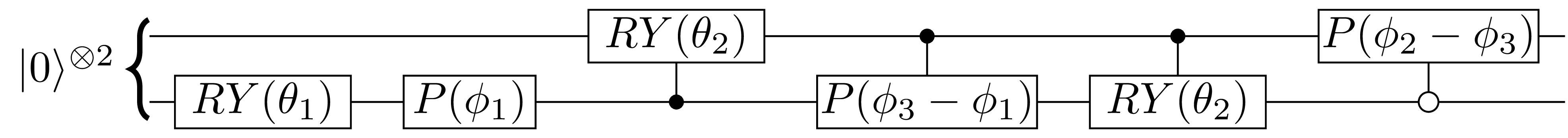Preparation of an arbitrary two-qubits state: we notice that we have 4 controlled operations which will be quite expensive.

The last negated control (change target if control is not set) can be replaced by a positive control (change target if control is set) by sandwiching the control between two $X$ gates. This replacement yields the following circuit: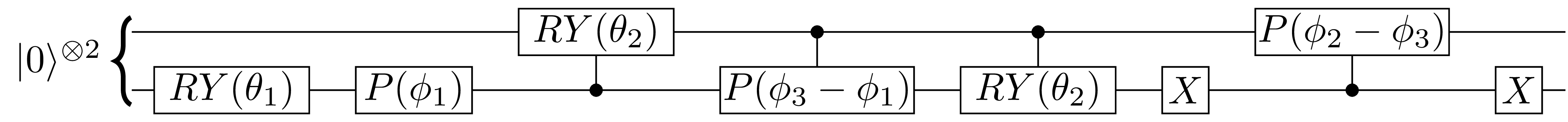Preparation of an arbitrary two-qubits state: we change the negative control to a positive control so the circuit is easy to work with in PennyLane.
The derived parametrized quantum circuit is inefficient
Notice that we require 4 controlled rotations that will amount to 8 controlled CNOTs and 8 single-qubit rotations. This is wildly inefficient.
This derivation is shown for completeness sake, there are better PQCs!
1. Nielsen, M. A., & Chuang, I. L. (2010). Quantum Computation and Quantum Information: 10th Anniversary Edition. Cambridge University Press. https://doi.org/10.1017/CBO9780511976667
2. Tilly, J., Chen, H., Cao, S., Picozzi, D., Setia, K., Li, Y., Grant, E., Wossnig, L., Rungger, I., Booth, G. H., & Tennyson, J. (2022). The Variational Quantum Eigensolver: A review of methods and best practices. Physics Reports, 986, 1–128. https://doi.org/10.1016/j.physrep.2022.08.003
3. Peruzzo, A., McClean, J., Shadbolt, P., Yung, M.-H., Zhou, X.-Q., Love, P. J., Aspuru-Guzik, A., & O’Brien, J. L. (2014). A variational eigenvalue solver on a photonic quantum processor. Nature Communications, 5(1). https://doi.org/10.1038/ncomms5213
4. Wecker, D., Hastings, M. B., & Troyer, M. (2015). Progress towards practical quantum variational algorithms. Physical Review A, 92(4). https://doi.org/10.1103/physreva.92.042303
5. Kandala, A., Mezzacapo, A., Temme, K., Takita, M., Brink, M., Chow, J. M., & Gambetta, J. M. (2017). Hardware-efficient variational quantum eigensolver for small molecules and quantum magnets. Nature, 549(7671), 242–246. https://doi.org/10.1038/nature23879
6. Murta, B., Cruz, P. M. Q., & Fernández-Rossier, J. (2023). Preparing valence-bond-solid states on noisy intermediate-scale quantum computers. Physical Review Research, 5(1). https://doi.org/10.1103/physrevresearch.5.013190
7. Shende, V. V., Markov, I. L., & Bullock, S. S. (2004). Minimal universal two-qubit controlled-NOT-based circuits. Physical Review A, 69(6). https://doi.org/10.1103/physreva.69.062321
8. Bonet-Monroig, X., Wang, H., Vermetten, D., Senjean, B., Moussa, C., Bäck, T., Dunjko, V., & O’Brien, T. E. (2023). Performance comparison of optimization methods on variational quantum algorithms. Physical Review A, 107(3). https://doi.org/10.1103/physreva.107.032407
9. Mitarai, K., Negoro, M., Kitagawa, M., & Fujii, K. (2018). Quantum circuit learning. Physical Review A, 98(3). https://doi.org/10.1103/physreva.98.032309
10. Kochenderfer, M. J., & Wheeler, T. A. (2019). Algorithms for Optimization. The MIT Press.
11. Gonthier, J. F., Radin, M. D., Buda, C., Doskocil, E. J., Abuan, C. M., & Romero, J. (2022). Measurements as a roadblock to near-term practical quantum advantage in chemistry: Resource analysis. Physical Review Research, 4(3). https://doi.org/10.1103/physrevresearch.4.033154
12. McClean, J. R., Boixo, S., Smelyanskiy, V. N., Babbush, R., & Neven, H. (2018). Barren plateaus in quantum neural network training landscapes. Nature Communications, 9(1). https://doi.org/10.1038/s41467-018-07090-4